# Half Past the Hour

Half Past the Hour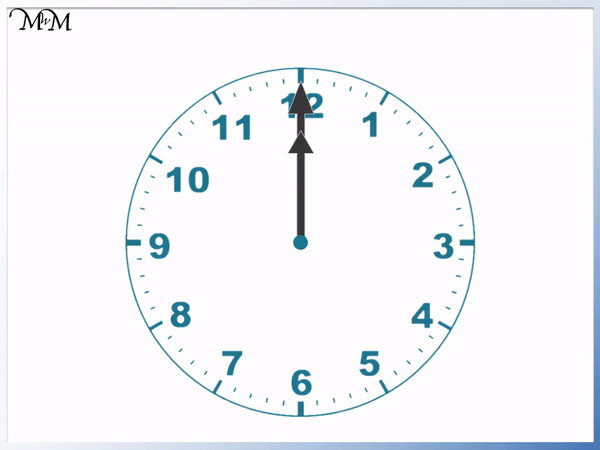• The larger minute hand completes a full turn in one hour.
• A new hour begins when the minute hand reaches the top, pointing at the 12.
• When the minute hand completes half of a full turn, half an hour has passed.
• It is half past the hour when the larger minute hand reaches the bottom, pointing at the 6.
• From 1 o’clock, the minute hand moves half way around to point at the 6.
• We call this time half past 1, because we are half way past 1 o’clock.
• We can see that the smaller hour hand is half way between the hours of 1 and 2 o’clock.
• Half past 1 is the time that is exactly half way between 1 and 2 o’clock.
• Because there are 60 minutes in an hour, 30 minutes have passed.
• The time shown is half past 1, which can also be written as 1:30 or 30 minutes past 1.

It is half past the hour when the larger minute hand is pointing to the 6.

We say it is half past the hour that the hour hand has just moved past.• The minute hand completes 8 full turns to get to 8 o’clock.
• At 8 o’clock, the hour hand points exactly to 8 and the minute hand points exactly to 12.
• The minute hand then completes another half turn.
• Because the minute hand is pointing at the 6, we know that the time is half past the hour.
• The time is half past 8.
• We know this because the hour hand has gone past the 8 but has not yet reached the 9.
• The hour hand is halfway between the 8 and the 9.Supporting Lessons# Reading Half Past the Hour an an Analogue Clock

It is important to understand the different parts of a clock face.

The longer hand is called the minutes hand. It completes a full turn every hour. This is 60 minutes in total.

The shorter hand is called the hour hand. It moves from one number on the clock face to the next every hour. The hour hand completes a full turn of the clock face twice each day.The number on the clock face that the hour hand points to tells us what hour it is. If the hour hand is not pointing exactly at a number, then the hour we are in is the number that the hour hand has just moved past.

We always look at the number that the hour hand has gone past already. We never look at the closest number to read what hour it is.

The minute hand tells us how many minutes past the hour it is. The small lines on the edge of the clock are the minutes.

## What is Half Past the Hour?

Half past the hour is when the minutes hand of a clock is pointing at the 6. This means that the minutes hand has completed half a turn, or half an hour since the last hour. 30 minutes have passed in half an hour.

Half past means 30 minutes past an hour. The number on the clock that the hour hand has just gone past tells us which hour we are half an hour past.

For example at half past one the larger minutes hand is pointing at the 6 and the smaller hour hand is halfway between the 1 and the 2.At the start of each new hour, the minutes hand starts at the top of the clock, pointing at 12. We can see that once it points at 6, it has travelled half way around the clock and half an hour has passed.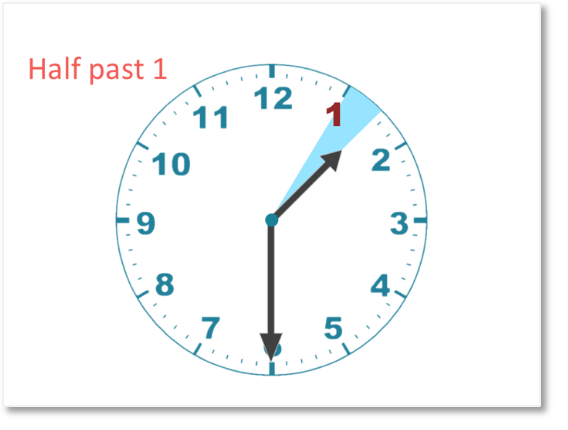We can see that the smaller hour hand has also completed half of its journey from 1 to 2.

We can tell if a time is half past the hour because the minutes hand will point at six and the hour hand will be exactly half way between two numbers on the clock face.

When teaching half past the hour, we need to make sure that we look at the hour we have gone past already. It is a common mistake for young children to say half past the wrong hour by reading the hour that it is coming up by mistake. We can say that it is always half past the smaller of the two numbers that the hand is in between.To read half past, look at the minute hand first. If the minute hand is pointing to the 6, then we know it is half past the hour.

Then look at the hour hand and look at the two numbers on the clock face that this hand is in between. The time is half past the smaller of these two numbers.

Because there are 60 minutes in a full hour, we can also read the time of half past as 30 minutes past.

In our example above, it is half past 1. We could say “30 minutes past 1” or we could even say “one thirty” for short.

## Teaching Half Past the Hour

When teaching half past the hour we can use the following steps:

• If the longer minutes hand points at the 6, then the time is half past the hour.
• Look at the shorter hour hand and read the two numbers on the clock face that this hand is in between.
• The time is half past the smaller of these two hours.
• The exception to this is when the hand is between 12 and 1, the time is half past 12.

Here is an example of telling the time to half past the hour.The minutes hand is pointing at the 6, so we know that the time is half past the hour.

We can see that the hour hand is in between 2 and 3.

2 is smaller than 3 and so, the time is half past 2.

Half past 2 can also be written as “30 minutes past two” or “two thirty”.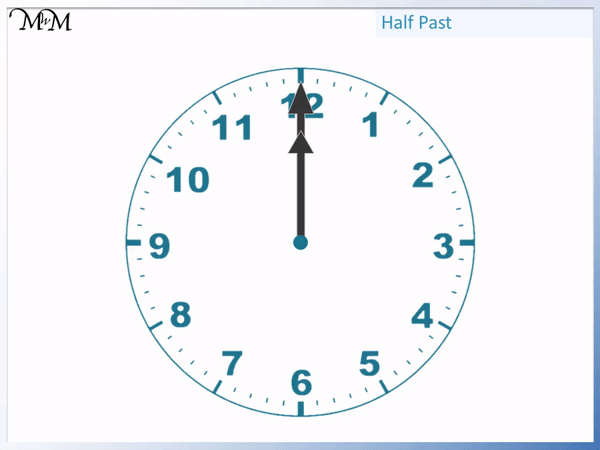We can see that at half past two, the hour hand has moved past the 2 and is exactly half way in between the 2 and the 3.

Here is another example of telling the time to half past the hour.

The longer minutes hand is pointing at the 6 so we know it is half past the hour.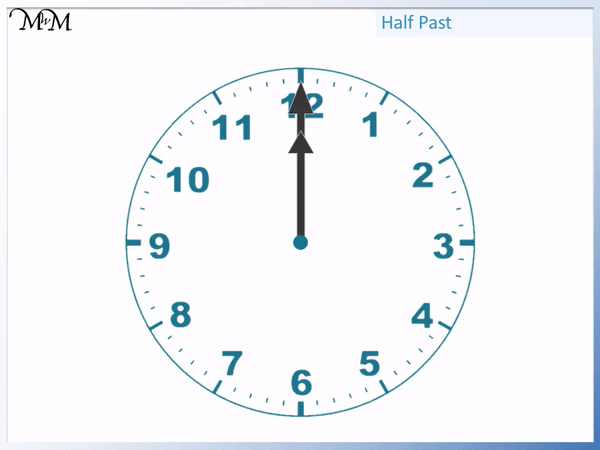The shorter hour hand is exactly in between the 8 and the 9.

8 is the smaller of these two numbers and so, the time is half past 8.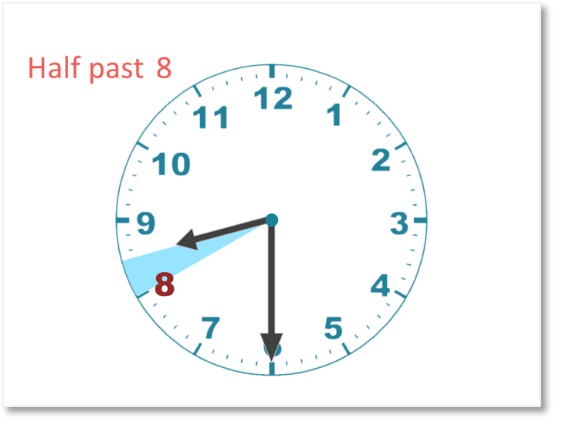Half past 8 can also be said as “30 minutes past 8′ or “8 thirty”. We can write this as 08:30.

Because the hour hand is half way past the 8, it is also half way towards the 9.

So at half past 8, there are 30 more minutes until 9 o’clock.

Here is another example. The minutes hand is pointing at the bottom, at 6, so we know that it is half past the hour.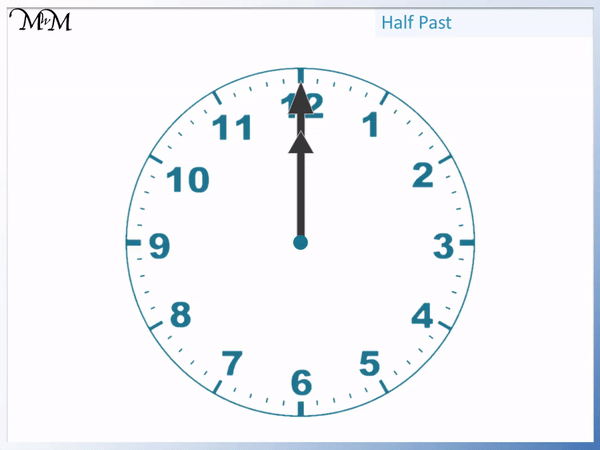The longer hand is pointing upwards between the 12 and the 1.

We have to be careful with our rule here. Even though the 12 is a larger number than 1, the hour hand travels past the 12 and towards the 1.

Here the 12 can be thought of as 0. When we start a new day, the hour hand begins at 12. On a digital clock, 12 o’clock in the morning of a new day can also be written as 00:00am. So we can think of the hour of 12 being like a 0.

So in this one exception to our rule, when the hour hand is exactly half way between 12 and 1, the time is half past 12.

We can also call this time “30 minutes past 12” or “12 thirty”.

With all of the examples above, an analogue clock does not tell us whether it is morning or night so we do not know if it is 12:30 am or 12:30 pm just by looking at the clock face. Fortunately we will already know if it is morning or afternoon!

The most challenging part of teaching telling the time to half past the hour is the child deciding the correct hour that we have gone past.

It helps to point at the hour hand with their finger and move it back anti-clockwise to see the humber that we have just gone past.

It helps to practise this skill regularly and you can use the random question generator link above to do this. I also recommend the worksheets above where you need to draw the hands on the clock to show each time. This is a harder skill than simply reading the time as it tests your ability to recognise exactly where the hands should be. This helps to embed the knowledge in their memory for longer.Now try our lesson on Telling the Time: Quarter Past the Hour where we learn how to read quarter past the hour on an analogue clock.error: Content is protected !!